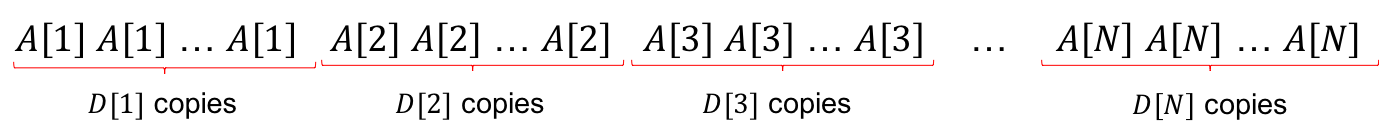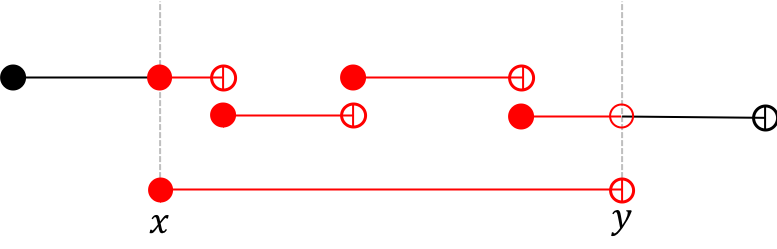Tester: Misha Chorniy
Editorialist: Suchan Park

EASY

Sorting

### PROBLEM:

There are a bunch of cards – it is known that there are D[i] cards that has an integer A[i] written.
Chef and Chefu are playing a game. There will be K rounds in total, Chef takes round 1,3,5,… (odd) and Chefu takes round 2,4,6,… (even). For the j-th round, the player must choose B[j] cards and discard all other cards. Chef wants to make sum of integers written the cards as large as possible, but Chefu wants it to be as small as possible. Assuming both players play optimally, what is the total sum of cards remaining at the end of the game?

### QUICK EXPLANATION:

Suppose all the cards are sorted in an array C: C, C, \cdots, C\Big[\sum_{i=1}^{n}D[i]\Big].

In each round, Chef will always take B[j] largest cards, and Chefu will always take B[j] smallest cards. Therefore in the end, the remaining cards form a segment [x, y) on the array C. We can compute x and y simply, so the problem reduces to calculating \sum_{k=x}^{y-1} C[k].

The reduced problem can be solved by sorting (A[i], D[i]) by A[i], and iterating through these pairs.

### EXPLANATION:

To solve game problems, we need to figure out what the best strategy is for each player. Each player has to choose B[j] cards among the available cards to maximize or minimize the sum of numbers on cards remaining at the end.

The instinct comes that each player must choose B[j] largest or smallest cards, respectively, to achieve the goal. And this is actually correct, because it is not always worse to replace any card with a bigger/smaller card.

So, it turned out the action of each player is actually quite simple!

Given a list of integers, what would you do if you were to choose some amount of largest or smallest integers? The most tractable solution is to just sort the input and extract the suffix and prefix, respectively. As these operations are exactly the same with the action of Chef and Chefu, we can do the exact same thing in this task, too.

It takes O(|\texttt{cards}| \log |\texttt{cards}|) time to simulate the action of each player, since we have to sort the whole sequence (which gives the time complexity) and then extract the suffix or prefix (linear time).

In this subtask, the total number of cards is no more than 1\,000 (since \sum_{i=1}^{N}D[i] = N \le 1000) and the number of operations is no more than 1\,000. If we just run the simulation K times, the time complexity will be O(K \cdot N \log N), which is enough.

To improve the time complexity a bit, we can use the fact that actually we don’t need to sort the whole sequence K times. This is because the prefix and the suffix of a sorted sequence is also sorted. If the sequence is already sorted, the simulation only takes O(N) time, since we just need to iterate over the prefix or the suffix.

So, we first sort the sequence in O(N \log N) (or possibly O(N^2), since N is small), and then run the O(N) simulation process K times, which gives time complexity O(N \log N + K \cdot N) or O(N^2 + K\cdot N).

Can we improve more? Yes!

Suppose all the cards are sorted in an array C: C, C, \cdots, C\Big[(\sum_{i=1}^{N}D[i])-1\Big]. Notice that Chef and Chefu both takes a substring of a sorted sequence. Therefore, at any time, the integers written on the remaining cards can be denoted as a substring of C. i.e. C[x], C[x+1], \cdots, C[y-1]. Note that this is an half-closed interval [x, y), and I use it because the number of elements inside the interval is y - x. We can analyze how each action changes the remaining interval:

• At the beginning, cards inside \Big[0, \sum_{i=1}^{N}D[i]\Big) are left.
• When it’s Chef’s turn, Chef takes the suffix of length B[j], so the interval becomes \Big[y - B[j], y\Big).
• When it’s Chefu’s turn, Chefu takes the prefix of length B[j], so the interval becomes \Big[x, x + B[j]\Big).

So, each operation takes constant time! Now we can solve this subtask in O(N \log N + K) or O(N^2 + K).

Here, \sum_{i=1}^{N}D[i] \le N \cdot 10^6 \le 10^{11}, so we don’t even afford to make the whole list.

But, we can still compute the left and right indices of the remaining cards in the array C using the process above, since the time complexity is just O(K). (doesn’t doesn’t depend on \sum_{i=1}^{N}D[i]) We just need to be aware that we have to use 64-bit integer types to store the indices of the endpoints.

Since the array C is given in the form “There are D[i] copies of the integer A[i]”, we can notice that there are lots of duplicate elements in the array. Sort (A[i], D[i]) pairs by nondecreasing order of A[i], so that A \le A \le \cdots \le A[N] holds. Then the array C will look like:For each i, we can find the indices of two endpoints of that particular group, which is \Big[\sum_{k=1}^{i-1}D[k], \sum_{k=1}^{i}D[k]\Big). This is because there are D + D + \cdots + D[i-1] elements in front of group i, and there are D[i] elements in group i. The picture below is an example when D = [5,3,4,6].Suppose cards inside [x, y) are left at the end of the game. Our goal is to compute the sum of elements inside this interval.From the picture above, we can see that each i contributes to the answer by

{\Large\{}(\texttt{length of the intersection between } [x, y) \texttt{ and } \Big[\sum_{k=1}^{i-1}D[k], \sum_{k=1}^{i}D[k]\Big){\Large\}} \times A[i]

because the number of occurences of A[i] in interval [x, y) is equal to the length of the intersecting segment.

Intersection of two half-closed segments [l_1, r_1) and [l_2, r_2) can be computed as {\Big[}\max(l_1, l_2), \min(r_1, r_2){\Big)}. This can be derived by

\begin{equation} \begin{split} [l_1, r_1) \cap [l_2, r_2) & = \{v:l_1 \le v < r_1\} \cap \{v:l_2 \le v < r_2\} \\ & = \{v:l_1 \le v\} \cap \{v: v < r_1\} \cap \{v :l_2 \le v\} \cap \{v: v < r_2\} \\ & = \{v:l_1 \le v\} \cap \{v :l_2 \le v\} \cap \{v: v < r_1\} \cap \{v: v < r_2\} \\ & = \{v:\max(l_1, l_2) \le v\} \cap \{v: v < \min(r_1, r_2)\} \\ & = \{v:\max(l_1, l_2) \le v < \min(r_1, r_2)\} \\ & = {\Big[}\max(l_1, l_2), \min(r_1, r_2){\Big)} \end{split} \end{equation}

Now, we can iterate all i and compute the intersection length, and add \texttt{length} \times A[i] to the answer. Note that we also need to use 64-bit integers here.

In total, the time complexity is O(N \log N), which is needed to sort all (A[i], D[i]) pairs.

### AUTHOR’S AND TESTER’S SOLUTIONS:

Author’s solution can be found here.
Tester’s solution can be found here.

### RELATED PROBLEMS:

2 Likes

What’s wrong with this solution of mine? Only passing first subtask

Can please someone explain me why my solution gets a TLE for subtask #2 while a similar solution gets AC with 0.02 sec time.
My Solution:
https://www.codechef.com/viewsolution/17540500

Other User’s Similar Solution:
https://www.codechef.com/viewsolution/17528051

Thank You.

Interesting.

My approach was to focus for each play on the number of cards discarded. Then I can total the discards made for each player, since they are entirely separate, and remove those blocks of cards from each end of the sorted rank-frequency array (a_i, d_i).

I was considering whether it’s worth calculating a cumulative frequency in order to reduce the work of finding the remaining card values (using a binary search). It would be an extra calculation pass through the array but without comparisons.